﻿ 高效直驱推进电机设计方法

# 高效直驱推进电机设计方法Design Method of High Efficiency Direct Drive Motor

Abstract: According to the characteristics of the propulsion, the external load characteristics of the high efficiency motor are analyzed, and the overall design of the propulsion motor is carried out with efficiency as the starting point. From the angle of iron loss, copper loss and harmonic loss, the optimization design of the motor is carried out to reduce the electromagnetic loss, and from the angle of bearing friction loss and rotor friction loss, the optimal design of motor loss reduction is carried out.

1. 引言

2. 负载特性分析

vp为螺旋桨的进速，单位为m/s；

vs为船舶的速度，单位为m/s；

ω为伴流系数；

D为螺旋桨直径，单位为m；

P为螺旋桨的推力，单位为N；

M为螺旋桨的转矩，单位为N∙m；

ρ为海水的密度，单位为kg/m3，通常取1025 kg/m3

$T\propto {n}^{2}$ (3)

n为电机转矩，单位为r/min。

${P}_{M}=Tn/9.55$ (4)

${P}_{M}\propto {n}^{3}\propto {T}^{2}$ (5)

3. 总体设计

200 kW级高效直驱推进电机的设计输入指标要求如表2所示。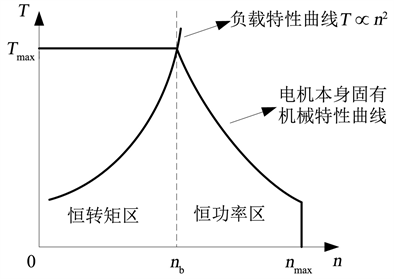Figure 1. Relation diagram of motor characteristics of mechanical and loadTable 1. Comparison between high efficiency direct drive motor and traditional propulsion plant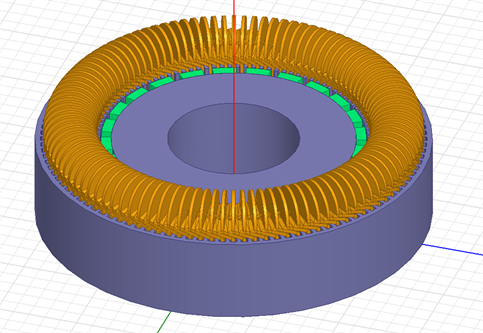Figure 2. Three dimensional electromagnetic simulation diagram of motor

4. 高效优化设计

1) 低电磁损耗优化设计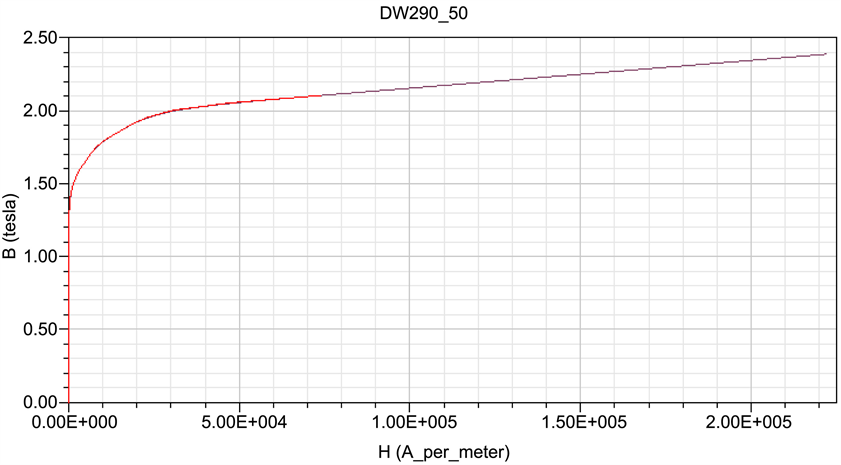Figure 3. Magnetization curve of 50W290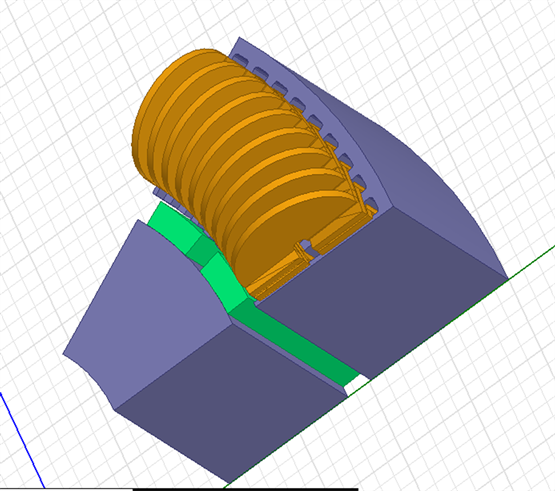Figure 4. Span diagram of motor windingTable 4. Copper loss comparison of two kinds of motor when the speed is 60 rpm

2) 低机械损耗优化设计

5. 小结Table 5. Comparison of harmonic loss of pole and non oblique pole motor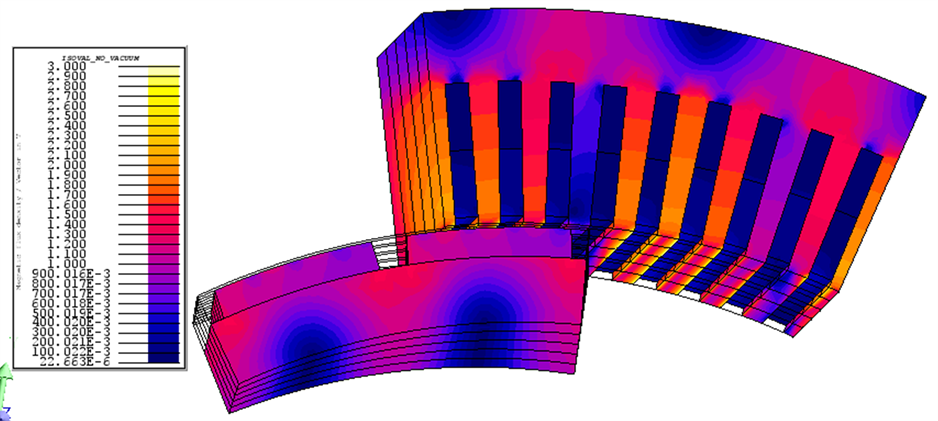Figure 5. Magnetic density distribution diagram of motor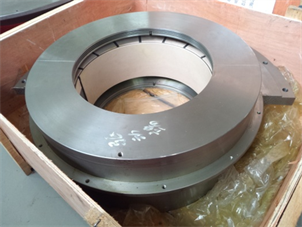Figure 6. Sliding bearing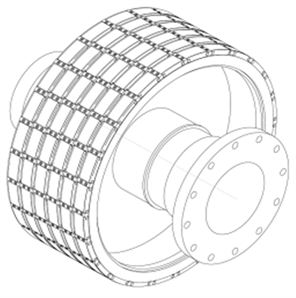Figure 7. Diagram of rotor structure

 高海波, 高孝洪, 陈辉. 船舶电力推进几种典型方式的比较[J]. 航海技术, 2006(6): 54-57.

 郭燚. 船舶电力推进双三相永磁同步电机驱动控制研究[D]: [博士学位论文]. 上海: 上海海事大学, 2006.

 刘梦觉, 耿鹏, 梁树甜. 螺旋桨负载的仿真数据管理技术分析[J]. 船电技术, 2016, 5(36): 8-11.

 陈益广, 潘玉玲, 贺鑫. 永磁同步电机分数槽集中绕组磁动势[J]. 电工技术学报, 2010, 25(10): 30-36.

 唐任远. 现代永磁电机理论与设计[M]. 北京: 机械工业出版社, 1997.

 许实章. 电机学[M]. 北京: 机械工业出版社, 1981.

 曾海景. 考虑圆度误差对滑动轴承转子系统摩擦功率损耗的影响[D]: [硕士学位论文]. 柳州: 广西工学院, 2012.

Top